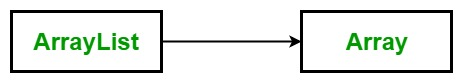GFG App
Open AppBrowser
Continue

# C# | How to convert an ArrayList to Array

In C#, an array is a group of like-typed variables that are referred to by a common name. And each data item is called an element of the array. The data types of the elements may be any valid data type like char, int, float etc. and the elements are stored in a contiguous location.
ArrayList represents an ordered collection of an object that can be indexed individually. It is basically an alternative to an array. It allows dynamic memory allocation, adding, searching and sorting items in the list.Below are the two methods for converting an ArrayList to an Array:
Method 1: Convert an ArrayList to Array of object
Syntax:

`public virtual object[] ToArray ();`

Explanation:

• It copy the elements of the ArrayList to a new Object array.
• It return an Object array containing copies of the elements of the ArrayList.

Example: In the below program, mylist is the ArrayList and we added 7 items to it which are the name of weekdays. Then we are creating an object array obj1 and using ToArray method with mylist we will assign the Arraylist elements to the object array. Finally, using foreach loop you can print the required converted array.

## CSharp

 `// C# program to illustrate ToArray() Method` `using` `System;` `using` `System.Collections;`   `class` `GFG {`   `    ``// Main Method` `    ``public` `static` `void` `Main()` `    ``{`   `        ``// Create and initializing ArrayList` `        ``ArrayList mylist = ``new` `ArrayList(7);`   `        ``mylist.Add(``"Monday"``);` `        ``mylist.Add(``"Tuesday"``);` `        ``mylist.Add(``"Wednesday"``);` `        ``mylist.Add(``"Thursday"``);` `        ``mylist.Add(``"Friday"``);` `        ``mylist.Add(``"Saturday"``);` `        ``mylist.Add(``"Sunday"``);`   `        ``// Copy the data of Arraylist into` `        ``// the object Array Using ToArray()` `        ``// method` `        ``object``[] obj1 = mylist.ToArray();`   `        ``foreach``(``string` `st ``in` `obj1)` `        ``{` `            ``Console.WriteLine(st);` `        ``}` `    ``}` `}`

Output:

```Monday
Tuesday
Wednesday
Thursday
Friday
Saturday
Sunday```

Method 2: Convert An ArrayList to Array of specified type
Syntax:

`public virtual Array ToArray (Type t);`

Here, t is the element Type of the destination array to create and copy elements.
Explanation:

• It copy the elements of the ArrayList to a new array of the specified element type.
• It return an array of the specified element type containing copies of the elements of the ArrayList.
• It throws ArgumentNullException if the value of t is null.
• It throws InvalidCastException if the type of the source ArrayList cannot be cast automatically to the specified type.

Example 1:

## CSharp

 `// C# program to illustrate the use ` `// of ToArray(Type) Method in the ` `// conversion of ArrayList to Array` `using` `System;` `using` `System.Collections;`   `class` `GFG {`   `    ``// Main Method` `    ``public` `static` `void` `Main()` `    ``{`   `        ``// Create and initialize new array` `        ``ArrayList mylist = ``new` `ArrayList(5);`   `        ``mylist.Add(``"Ruby"``);` `        ``mylist.Add(``"C#"``);` `        ``mylist.Add(``"C++"``);` `        ``mylist.Add(``"Java"``);` `        ``mylist.Add(``"Perl"``);`   `        ``// Copy the data of Arraylist into` `        ``// the string Array Using` `        ``// ToArray(Type) method` `        ``string``[] str = (``string``[])mylist.ToArray(``typeof``(``string``));`   `        ``// Display the data of str string` `        ``foreach``(``string` `j ``in` `str)` `        ``{` `            ``Console.WriteLine(j);` `        ``}` `    ``}` `}`

Output:

```Ruby
C#
C++
Java
Perl```

Explanation of Code: Here, we are converting an ArrayList to Array of specified type i.e string type. For conversion, you have to use ToArray(Type) method along with typeof keyword. And then you have to explicitly cast it to the specified type. Here you can see the line of code, string[] str = (string[])mylist.ToArray(typeof(string));. (string[]) is used for mylist to convert it to string array type. There is another alternative for this line of code as shown in below example.
Example 2:

## CSharp

 `// C# program to illustrate the use ` `// of ToArray(Type) Method in the ` `// conversion of ArrayList to Array` `using` `System;` `using` `System.Collections;`   `class` `GFG {`   `    ``// Main Method` `    ``public` `static` `void` `Main()` `    ``{`   `        ``// Create and initialize new array` `        ``ArrayList mylist = ``new` `ArrayList(5);`   `        ``mylist.Add(``"Ruby"``);` `        ``mylist.Add(``"C#"``);` `        ``mylist.Add(``"C++"``);` `        ``mylist.Add(``"Java"``);` `        ``mylist.Add(``"Perl"``);`   `        ``// Copy the data of Arraylist into` `        ``// the string Array Using` `        ``// ToArray(Type) method` `        ``// see this clearly as here we ` `        ``// are using as keyword` `        ``string``[] str = mylist.ToArray(``typeof``(``string``)) ``as` `string``[];`   `        ``// Display the data of str string` `        ``foreach``(``string` `j ``in` `str)` `        ``{` `            ``Console.WriteLine(j);` `        ``}` `    ``}` `}`

Output:

```Ruby
C#
C++
Java
Perl```

#### What will happen if the ArrayList doesn’t contain all the elements of the same type?

If the ArrayList doesn’t contain the elements of the same type then your conversion(ArrayList to Array) will throw InvalidCastException.
Example:

## CSharp

 `// C# program to illustrate the use ` `// of ToArray(Type) Method in the ` `// conversion of ArrayList to Array` `using` `System;` `using` `System.Collections;` ` `  `class` `GFG {` ` `  `    ``// Main Method` `    ``public` `static` `void` `Main()` `    ``{` ` `  `        ``// Create and initialize new array` `        ``ArrayList mylist = ``new` `ArrayList(5);` ` `  `        ``mylist.Add(``"Ruby"``);` `        ``mylist.Add(5);` `        ``mylist.Add(``"C++"``);` `        ``mylist.Add(7);` `        ``mylist.Add(``"Perl"``);` ` `  `        ``// It will throw the InvalidCastException` `        ``// Copy the data of Arraylist into` `        ``// the string Array Using` `        ``// ToArray(Type) method` `        ``string``[] str = mylist.ToArray(``typeof``(``string``)) ``as` `string``[];` ` `  `        ``// Display the data of str string` `        ``foreach``(``string` `j ``in` `str)` `        ``{` `            ``Console.WriteLine(j);` `        ``}` `    ``}` `}`

Runtime Error:

Unhandled Exception:
System.InvalidCastException: Specified cast is not valid.

My Personal Notes arrow_drop_up